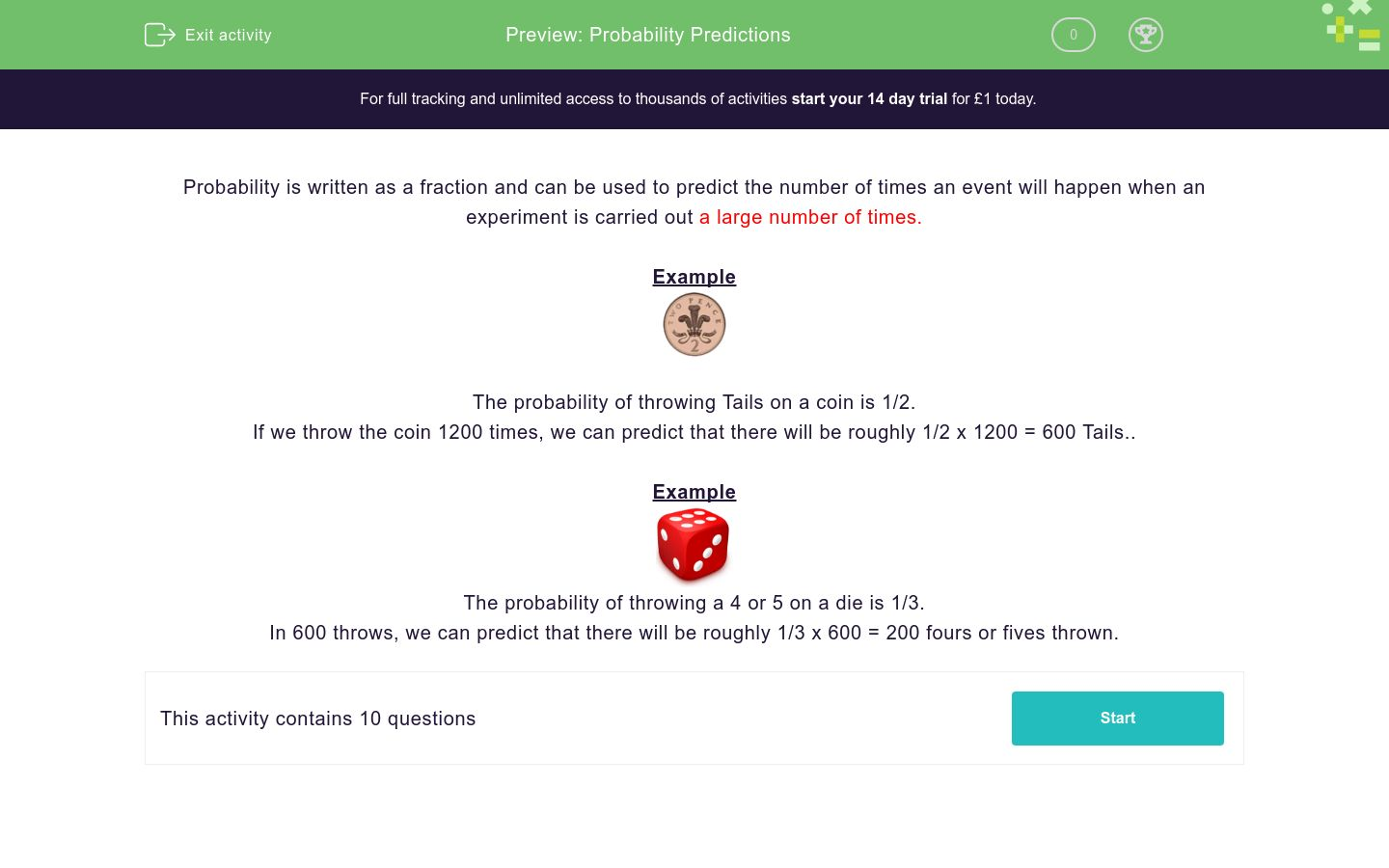# Probability Predictions

In this worksheet, students use probability to predict how often an event is likely to happen.Key stage:  KS 3

Curriculum topic:  Probability

Curriculum subtopic:  Understand Probability Outcomes

Difficulty level:### QUESTION 1 of 10

Probability is written as a fraction and can be used to predict the number of times an event will happen when an experiment is carried out a large number of times.

ExampleThe probability of throwing Tails on a coin is 1/2.

If we throw the coin 1200 times, we can predict that there will be roughly 1/2 x 1200 = 600 Tails..

ExampleThe probability of throwing a 4 or 5 on a die is 1/3.

In 600 throws, we can predict that there will be roughly 1/3 x 600 = 200 fours or fives thrown.If we throw this fair coin 1000 times, roughly predict how many times you would expect to get Tails.If we throw this fair coin 5000 times, roughly predict how many times you would expect to get Heads.If we throw this fair die 6000 times, roughly predict how many times you would expect to get a six.If we throw this fair die 6000 times, roughly predict how many times you would expect to get a one.If we throw this fair die 6000 times, roughly predict how many times you would expect to get an even number.If we throw this fair die 6000 times, roughly predict how many times you would expect to get a ten.If we spin this fair spinner 800 times, roughly predict how many times you would expect it to land on green.If we spin this fair spinner 800 times, roughly predict how many times you would expect it to land on yellow.If we spin this fair spinner 1600 times, roughly predict how many times you would expect it to land on yellow.If we spin this fair spinner 2400 times, roughly predict how many times you would expect it to land on green.

• Question 1If we throw this fair coin 1000 times, roughly predict how many times you would expect to get Tails.

500
• Question 2If we throw this fair coin 5000 times, roughly predict how many times you would expect to get Heads.

2500
• Question 3If we throw this fair die 6000 times, roughly predict how many times you would expect to get a six.

1000
• Question 4If we throw this fair die 6000 times, roughly predict how many times you would expect to get a one.

1000
• Question 5If we throw this fair die 6000 times, roughly predict how many times you would expect to get an even number.

3000
• Question 6If we throw this fair die 6000 times, roughly predict how many times you would expect to get a ten.

0
• Question 7If we spin this fair spinner 800 times, roughly predict how many times you would expect it to land on green.

200
• Question 8If we spin this fair spinner 800 times, roughly predict how many times you would expect it to land on yellow.

300
• Question 9If we spin this fair spinner 1600 times, roughly predict how many times you would expect it to land on yellow.

600
• Question 10If we spin this fair spinner 2400 times, roughly predict how many times you would expect it to land on green.